Related Articles

# Swarmplot using Seaborn in Python

• Last Updated : 06 Nov, 2020

Seaborn is an amazing visualization library for statistical graphics plotting in Python. It provides beautiful default styles and color palettes to make statistical plots more attractive. It is built on the top of the matplotlib library and also closely integrated into the data structures from pandas.

## Swarm Plot

Seaborn swarmplot is probably similar to stripplot, only the points are adjusted so it won’t get overlap to each other as it helps to represent the better representation of the distribution of values. A swarm plot can be drawn on its own, but it is also a good complement to a box, preferable because the associated names will be used to annotate the axes. This type of plot sometimes known as “beeswarm”.

Syntax: seaborn.swarmplot(x=None, y=None, hue=None, data=None, order=None, hue_order=None, dodge=False, orient=None, color=None, palette=None, size=5, edgecolor=’gray’, linewidth=0, ax=None, **kwargs)

Parameters:
x, y, hue: Inputs for plotting long-form data.
data: Dataset for plotting.
color: Color for all of the elements
size: Radius of the markers, in points.

Example 1: Basic visualization of “fmri” dataset using swarmplot()

## Python3

 `import` `seaborn`  `seaborn.``set``(style``=``'whitegrid'``)``fmri ``=` `seaborn.load_dataset(``"fmri"``)` `seaborn.swarmplot(x``=``"timepoint"``,``                  ``y``=``"signal"``,``                  ``data``=``fmri)`

Output: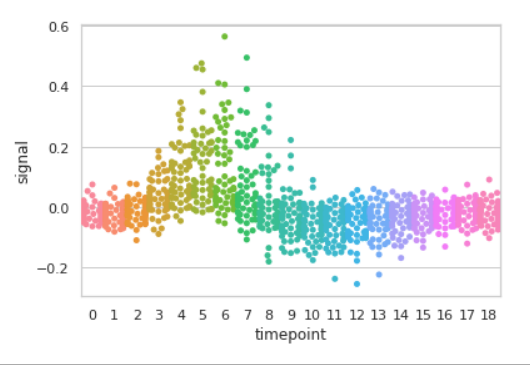Example 2: Grouping data points on the basis of category, here as region and event.

## Python3

 `import` `seaborn`  `seaborn.``set``(style``=``'whitegrid'``)``fmri ``=` `seaborn.load_dataset(``"fmri"``)` `seaborn.swarmplot(x``=``"timepoint"``,``                  ``y``=``"signal"``,``                  ``hue``=``"region"``,``                  ``data``=``fmri)`

Output: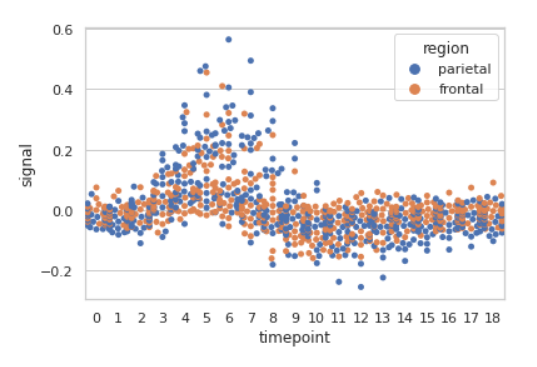Example 3: Basic visualization of “tips” dataset using swarmplot()

## Python3

 `import` `seaborn`  `seaborn.``set``(style``=``'whitegrid'``)``tip ``=` `seaborn.load_dataset(``'tips'``)` `seaborn.swarmplot(x``=``'day'``, y``=``'tip'``, data``=``tip)`

Output: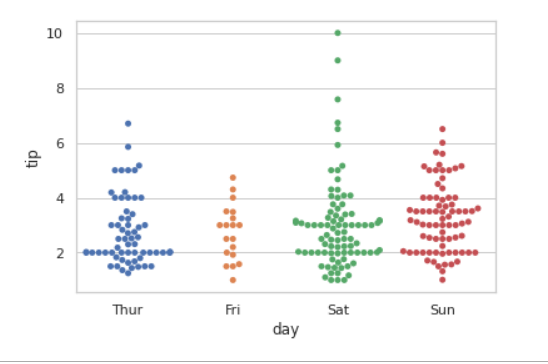## Grouping variables in Seaborn Swarmplot with different attributes

1. Draw a single horizontal swarm plot using only one axis:

If we use only one data variable instead of two data variables then it means that the axis denotes each of these data variables as an axis.

X denotes an x-axis and y denote a y-axis.

Syntax:

```seaborn.swarmplot(x)
```

## Python3

 `# Python program to illustrate``# swarmplot using inbuilt data-set``# given in seaborn`` ` `# importing the required module``import` `seaborn` `# use to set style of background of plot``seaborn.``set``(style``=``"whitegrid"``)` `# loading data-set``tips ``=` `seaborn.load_dataset(``"tips"``)` `seaborn.swarmplot(x``=``tips[``"total_bill"``])`

Output: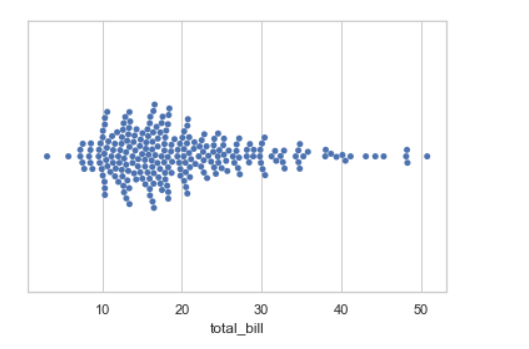2. Draw horizontal swarms:

In the above example we see how to plot single horizontal swarm plot and here can perform multiple horizontal swarm plot with exchange the data variable with another axis.

## Python3

 `# Python program to illustrate``# swarmplot using inbuilt data-set``# given in seaborn`` ` `# importing the required module``import` `seaborn` `# use to set style of background of plot``seaborn.``set``(style``=``"whitegrid"``)` `# loading data-set``tips ``=` `seaborn.load_dataset(``"tips"``)` `seaborn.swarmplot(x``=``"total_bill"``, y``=``"day"``, data``=``tips)`

Output: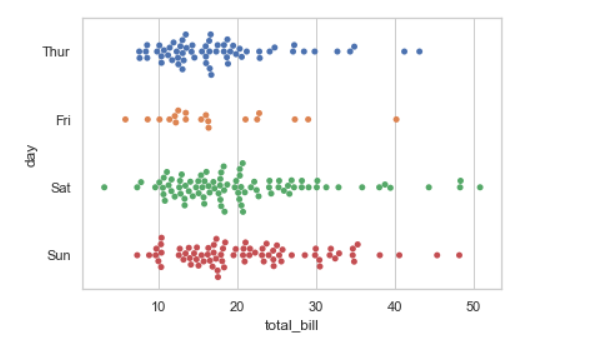3. Using hue parameter:

While the points are plotted in two dimensions, another dimension can be added to the plot by coloring the points according to a third variable.

Syntax:

sns.swarmplot(x, y, hue, data);

## Python3

 `# Python program to illustrate``# swarmplot using inbuilt data-set``# given in seaborn`` ` `# importing the required module``import` `seaborn` `# use to set style of background of plot``seaborn.``set``(style``=``"whitegrid"``)` `# loading data-set``tips ``=` `seaborn.load_dataset(``"tips"``)` `seaborn.swarmplot(x``=``"day"``, y``=``"total_bill"``, hue``=``"time"``, data``=``tips)`

Output: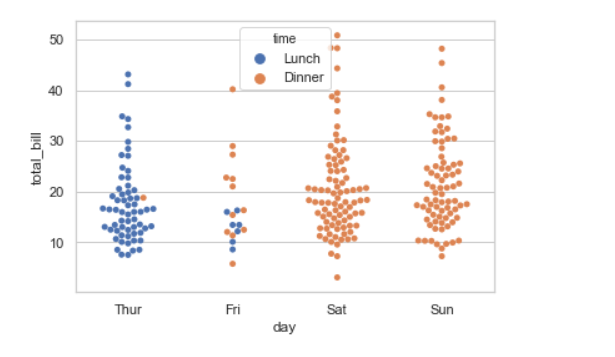4. Draw outlines around the data points using linewidth:

Width of the gray lines that frame the plot elements. Whenever we increase linewidth than the point also will increase automatically.

Syntax:

seaborn.swarmplot(x, y, data, linewidth)

## Python3

 `# Python program to illustrate``# swarmplot using inbuilt data-set``# given in seaborn`` ` `# importing the required module``import` `seaborn` `# use to set style of background of plot``seaborn.``set``(style``=``"whitegrid"``)` `# loading data-set``tips ``=` `seaborn.load_dataset(``"tips"``)` `seaborn.swarmplot(x``=``"day"``, y``=``"total_bill"``, data``=``tips,``                   ``linewidth``=``2``)`

Output: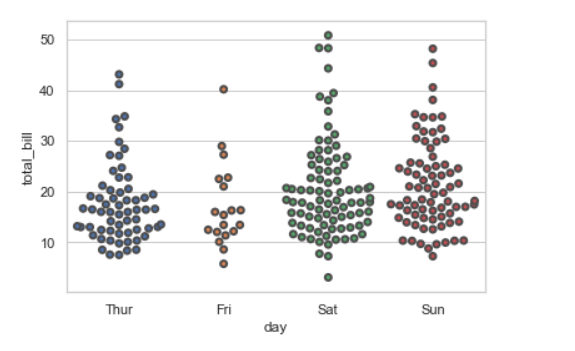We can change the color with edgecolor:

## Python3

 `seaborn.swarmplot(y``=``"total_bill"``, x``=``"day"``, data``=``tips,``                   ``linewidth``=``2``,edgecolor``=``'green'``)`

Output: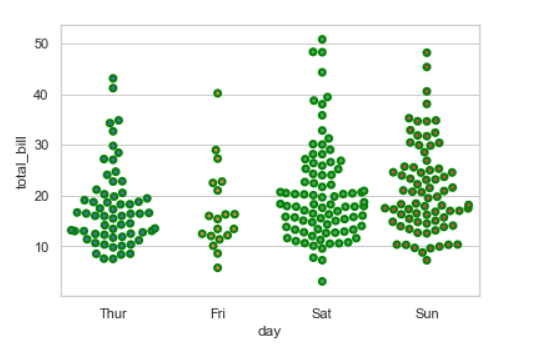5. Draw each level of the hue variable at different locations on the major categorical axis:

When using hue nesting, setting dodge should be True will separate the point for different hue levels along the categorical axis. And Palette is used for the different levels of the hue variable.

Syntax:

seaborn.stripplot(x, y, data, hue, palette, dodge)

## Python3

 `# Python program to illustrate``# swarmplot using inbuilt data-set``# given in seaborn`` ` `# importing the required module``import` `seaborn` `# use to set style of background of plot``seaborn.``set``(style``=``"whitegrid"``)` `# loading data-set``tips ``=` `seaborn.load_dataset(``"tips"``)` `seaborn.swarmplot(x``=``"day"``, y``=``"total_bill"``, hue``=``"smoker"``,``                   ``data``=``tips, palette``=``"Set2"``, dodge``=``True``)`

Output: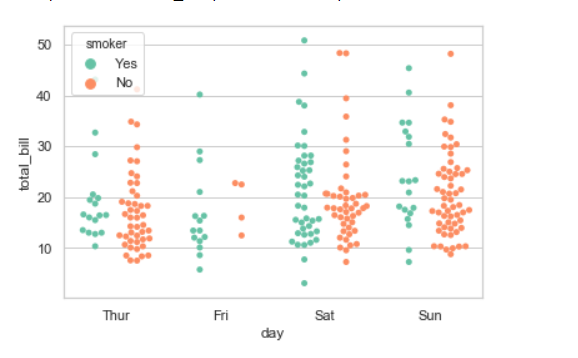Possible values of palette are:

Accent, Accent_r, Blues, Blues_r, BrBG, BrBG_r, BuGn, BuGn_r, BuPu, BuPu_r, CMRmap, CMRmap_r, Dark2, Dark2_r,

GnBu, GnBu_r, Greens, Greens_r, Greys, Greys_r, OrRd, OrRd_r, Oranges, Oranges_r, PRGn, PRGn_r, Paired, Paired_r,

Pastel1, Pastel1_r, Pastel2, Pastel2_r, PiYG, PiYG_r, PuBu, PuBuGn, PuBuGn_r, PuBu_r, PuOr, PuOr_r, PuRd, PuRd_r,

Purples, Purples_r, RdBu, RdBu_r, RdGy, RdGy_r, RdPu, RdPu_r, RdYlBu, RdYlBu_r, RdYlGn, RdYlGn_r, Reds, Reds_r, Set1,

Set1_r, Set2, Set2_r, Set3, Set3_r, Spectral, Spectral_r, Wistia, Wistia_r, YlGn, YlGnBu, YlGnBu_r, YlGn_r, YlOrBr,

YlOrBr_r, YlOrRd, YlOrRd_r, afmhot, afmhot_r, autumn, autumn_r, binary, binary_r, bone, bone_r, brg, brg_r, bwr, bwr_r,

cividis, cividis_r, cool, cool_r, coolwarm, coolwarm_r, copper, copper_r, cubehelix, cubehelix_r, flag, flag_r, gist_earth,

gist_earth_r, gist_gray, gist_gray_r, gist_heat, gist_heat_r, gist_ncar, gist_ncar_r, gist_rainbow, gist_rainbow_r, gist_stern,

6. Plotting large points and different aesthetics With marker and alpha parameter:

We will use alpha to manage transparency of the data point, and use marker for marker to customize the data point.

## Python3

 `# Python program to illustrate``# swarmplot using inbuilt data-set``# given in seaborn`` ` `# importing the required module``import` `seaborn` `# use to set style of background of plot``seaborn.``set``(style``=``"whitegrid"``)` `# loading data-set``tips ``=` `seaborn.load_dataset(``"tips"``)` `seaborn.swarmplot(x``=``"day"``, y``=``"total_bill"``, hue``=``"smoker"``,``                   ``data``=``tips, palette``=``"Set2"``, size``=``20``, marker``=``"D"``,``                   ``edgecolor``=``"gray"``, alpha``=``.``25``)`

Output: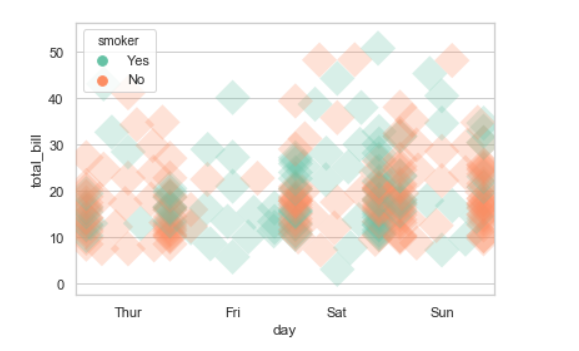7. Control swarm order by passing an explicit order:

## Python3

 `# Python program to illustrate``# swarmplot using inbuilt data-set``# given in seaborn`` ` `# importing the required module``import` `seaborn` `# use to set style of background of plot``seaborn.``set``(style``=``"whitegrid"``)` `# loading data-set``tips ``=` `seaborn.load_dataset(``"tips"``)` `seaborn.swarmplot(x``=``"time"``, y``=``"tip"``, data``=``tips,``                   ``order``=``[``"Dinner"``, ``"Lunch"``])`

Output: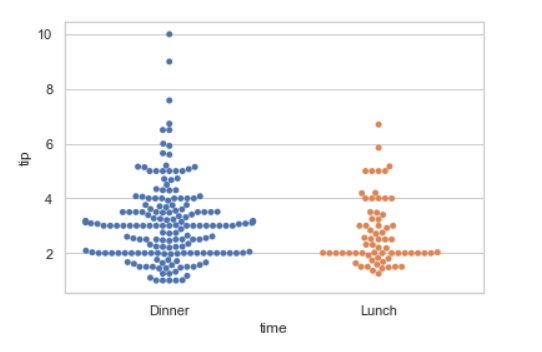Using size we can generate the point and we can produce points with different sizes.

Syntax:

seaborn.swramplot( x, y, data, size)

## Python3

 `# Python program to illustrate``# swarmplot using inbuilt data-set``# given in seaborn` `# importing the required module``import` `seaborn` `# use to set style of background of plot``seaborn.``set``(style``=``"whitegrid"``)` `# loading data-set``tips ``=` `seaborn.load_dataset(``"tips"``)` `seaborn.swarmplot(x``=``'day'``, y``=``'total_bill'``, data``=``tips,``                  ``hue``=``'smoker'``, size``=``10``)`

Output: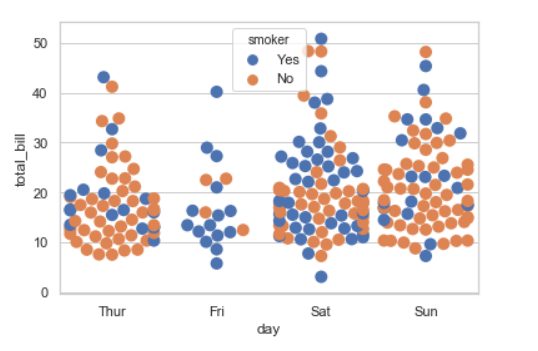Using the palette we can generate the point with different colors. In this below example we can see the palette can be responsible for a generate the swramplot with different colormap values.

Syntax:

seaborn.swramplot( x, y, data, palette=”color_name”)

## Python3

 `# Python program to illustrate``# swarmplot using inbuilt data-set``# given in seaborn` `# importing the required module``import` `seaborn` `# use to set style of background of plot``seaborn.``set``(style``=``"whitegrid"``)` `# loading data-set``tips ``=` `seaborn.load_dataset(``"tips"``)` `seaborn.swarmplot(x``=``'day'``, y``=``'total_bill'``, data``=``tips,``                  ``hue``=``'time'``, palette``=``'pastel'``)`

Output: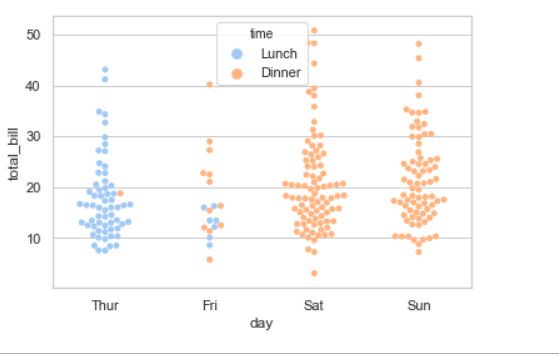Attention geek! Strengthen your foundations with the Python Programming Foundation Course and learn the basics.

To begin with, your interview preparations Enhance your Data Structures concepts with the Python DS Course. And to begin with your Machine Learning Journey, join the Machine Learning – Basic Level Course

My Personal Notes arrow_drop_up